Community ·TensorFlow with MATLAB
марта 14, 2023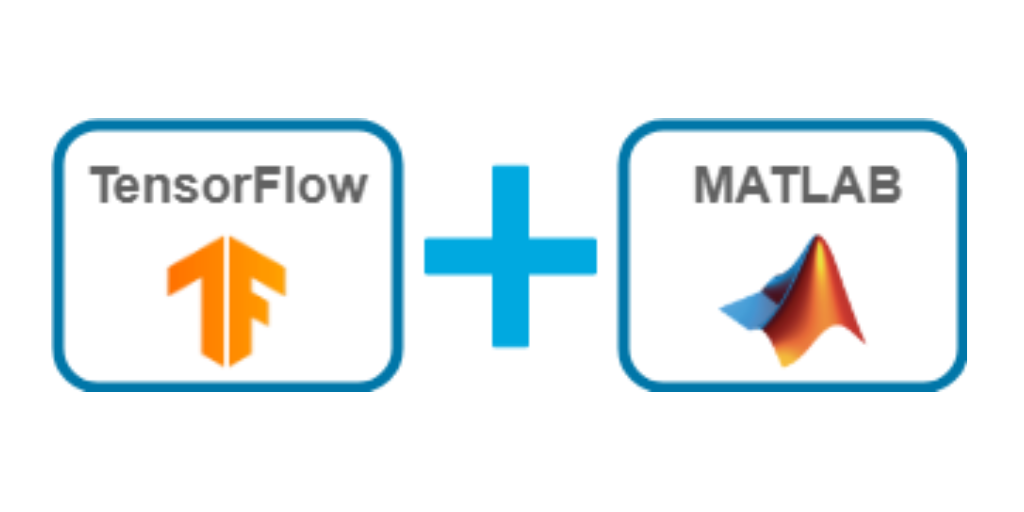Posted by Sivylla Paraskevopoulou, Product Marketing Manager at MathWorks

In this blog post I will show you how to use TensorFlow™ with MATLAB® for deep learning applications. More specifically, I will show you how to convert pretrained TensorFlow models to MATLAB models, convert models from MATLAB to TensorFlow, and use MATLAB and TensorFlow together.

These interoperability features, offered by MATLAB, enable collaboration between colleagues, teams, and communities that work on different platforms. Today’s post will show you how to use these features, and give you examples of when you might want to use them and how they connect the work of AI developers and engineers to enable domain-specific AI system design.

# Introduction

#### What is MATLAB?

MATLAB is a computing platform tailored for engineering and scientific applications like data analysis, signal and image processing, control systems, wireless communications, and robotics. MATLAB includes a programming language, interactive apps, and tools for automatically generating embedded code. MATLAB is also the foundation for Simulink®, a block diagram environment for simulating complex multi-domain systems.

Similarly to Python® libraries, MATLAB provides toolboxes for achieving different goals. More specifically, MATLAB provides the Deep Learning Toolbox™ for deep learning workflows. Deep Learning Toolbox provides a framework for designing and implementing deep neural networks with algorithms, pretrained models, and apps. It can be combined with domain-specific toolboxes in areas such as computer vision, signal processing, and audio applications.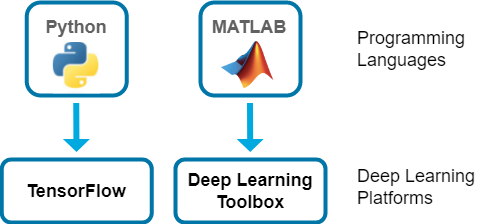Figure:Python and MATLAB are programming languages; Python can leverage the TensorFlow library for deep learning workflows, while MATLAB provides the Deep Learning Toolbox.

#### Why TensorFlow and MATLAB?

Both TensorFlow and MATLAB are widely used for deep learning. Many MATLAB customers are interested in integrating TensorFlow models into their AI design, for creating customized tools, simulating complex systems, or optimizing data modeling. TensorFlow users can also leverage MATLAB to generate, analyze, and visualize training data, post-process model output, and deploy trained neural networks to desktop, web apps, or embedded hardware.

For example, engineers have integrated TensorFlow models into Simulink (MATLAB simulation environment) to develop a battery state-of charge estimator for an electric vehicle and scientists have used MATLAB with TensorFlow to build a custom toolbox for reading climate data. For more details on these examples, see Integrate TensorFlow Model into Simulink for Simulation and Code Generation and Climate Data Store Toolbox for MATLAB.

#### What’s Next?

Now you have started to see the benefits of using TensorFlow with MATLAB. Let’s get into more of the technical details on how to use TensorFlow with MATLAB in the following three sections.

You will see how straightforward it is to use TensorFlow with MATLAB and why I (and other engineers) like having the option to combine them for deep learning applications. Why choose when you don’t have to?

# Convert Model from TensorFlow to MATLAB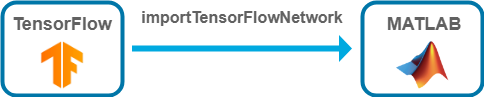You can convert a pretrained model from TensorFlow to MATLAB by using the MATLAB function importTensorFlowNetwork. A scenario when this function might be useful; a data scientist creates a model in TensorFlow and then an engineer integrates this model into an AI system created in MATLAB.

We will show you here how to import an image classification TensorFlow model into MATLAB and (1) use it for prediction and (2) integrate it into an AI system.

Convert model from TensorFlow to MATLAB
Before importing a pretrained TensorFlow model into MATLAB network, you must save the TensorFlow model in the SavedModel format.

Python code:

 ```import tensorflow as tf tf.saved_model.save(model.modelFolder) ```

Then, you can import the TensorFlow model into MATLAB by using the MATLAB function importTensorFlowNetwork. You only need one line of code!

MATLAB code:

 ```modelFolder = “EfficientNetV2L”; net = importTensorFlowNetwork(modelFolder,OutputLayerType=”classification”) ```

Classify Image
Read the image you want to classify. Resize the image to the input size of the network.
MATLAB code:
 ```Im = imread(“mydoc.jpg”); InputSize = net.Layers(1).InputSize; Im = imresize(Im,InputSize(1:2));```

Before you classify the image, the image might require further preprocessing or changing the dimension ordering from TensorFlow to MATLAB. To learn more and get answers to common questions about importing models, see Tips on Importing Models from TensorFlow.

Predict and plot image with classified label. MATLAB code:

 ```label = classify(net,Im); imshow(Im) title("Predicted label: " + string(label));```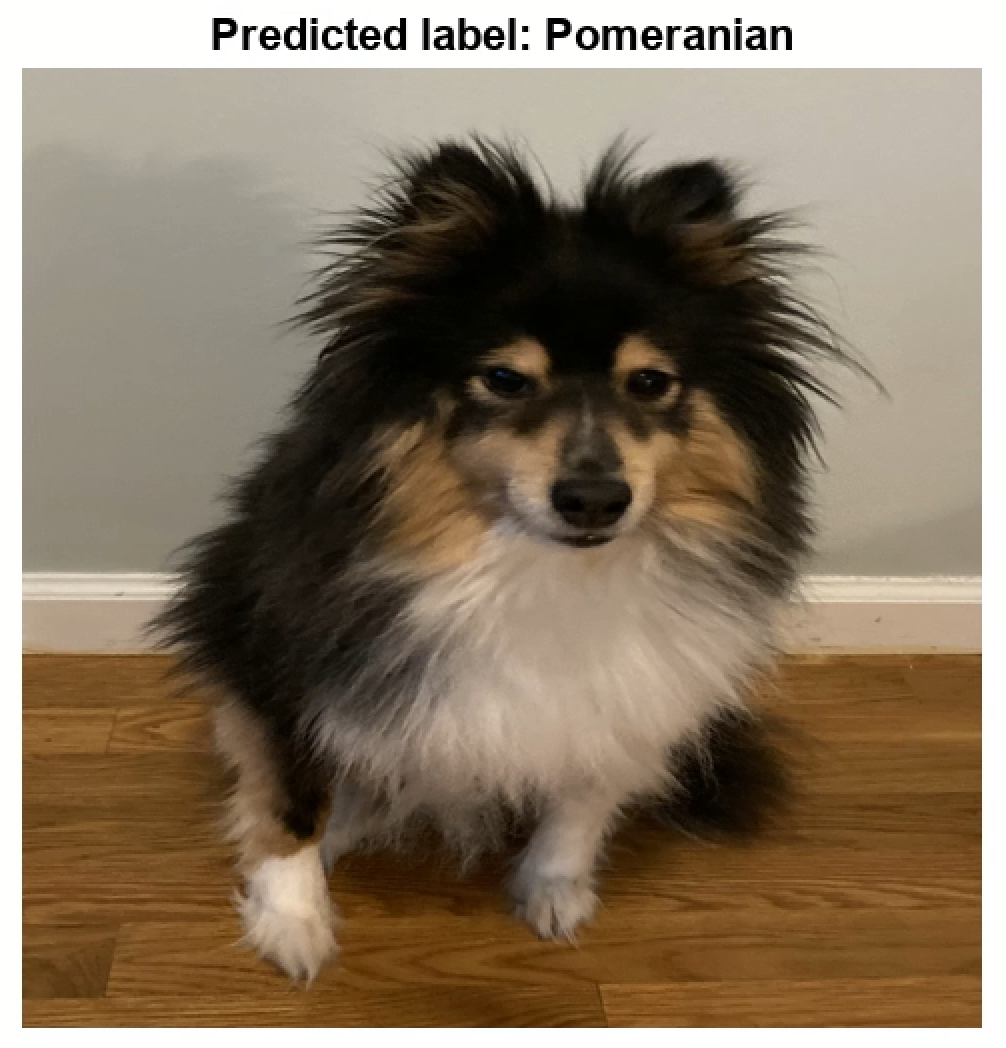To see the full example on how to import an image classification TensorFlow model into MATLAB and use the model for prediction, see Image Classification in MATLAB Using Converted TensorFlow Model. To learn more on importing TensorFlow models into MATLAB, check out the blog post Importing Models from TensorFlow, PyTorch, and ONNX.

Transfer Learning
A common reason to import a pretrained TensorFlow model into MATLAB is to perform transfer learning. Transfer learning is the process of taking a pretrained deep learning model and fine-tuning to fit the model to a new problem. For example, you are doing object detection in MATLAB, and you find a TensorFlow model that can improve the detection accuracy, but you need to retrain the model with your data. Using transfer learning is usually faster and easier than training a network from scratch.

In MATLAB, you can perform transfer learning programmatically or interactively by using the Deep Network Designer (DND) app. It’s easy to do model surgery (prepare a network to train on new data) with a few lines of MATLAB code by using built-in functions that replace, remove, or add layers at any part of the network architecture. For an example, see Train Deep Learning Network to Classify New Images. With DND, you can interactively prepare the network for training, train the network, export the retrained network, and then use it for the new task. For an example, see Transfer Learning with Deep Network Designer.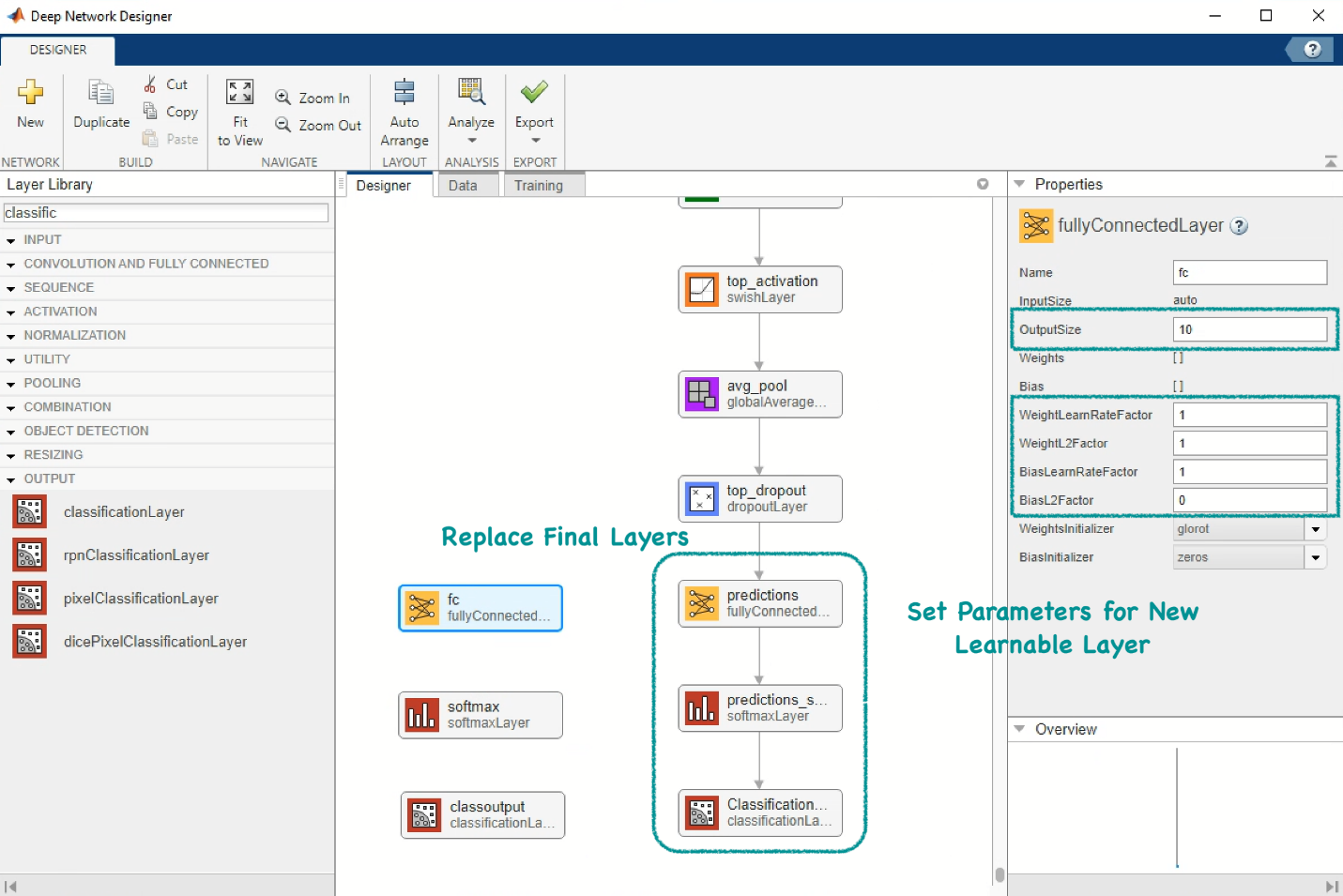Figure:Edit pretrained model with a low-code app for transfer learning.

Simulink is a block diagram environment used to design systems with multi-domain models, simulate systems before moving to hardware, and deploy without writing code. Simulink users have expressed interest in the ability to bring in AI models and simulate entire systems. In fact, this is very easy to do with Simulink blocks.

In the following figure, you can see a very simple AI system that reads and classifies an image using an imported TensorFlow model. Essentially, the Simulink system executes the same workflow shown above. To learn more about how to design and simulate such a system, see Classify Images in Simulink with Imported TensorFlow Network.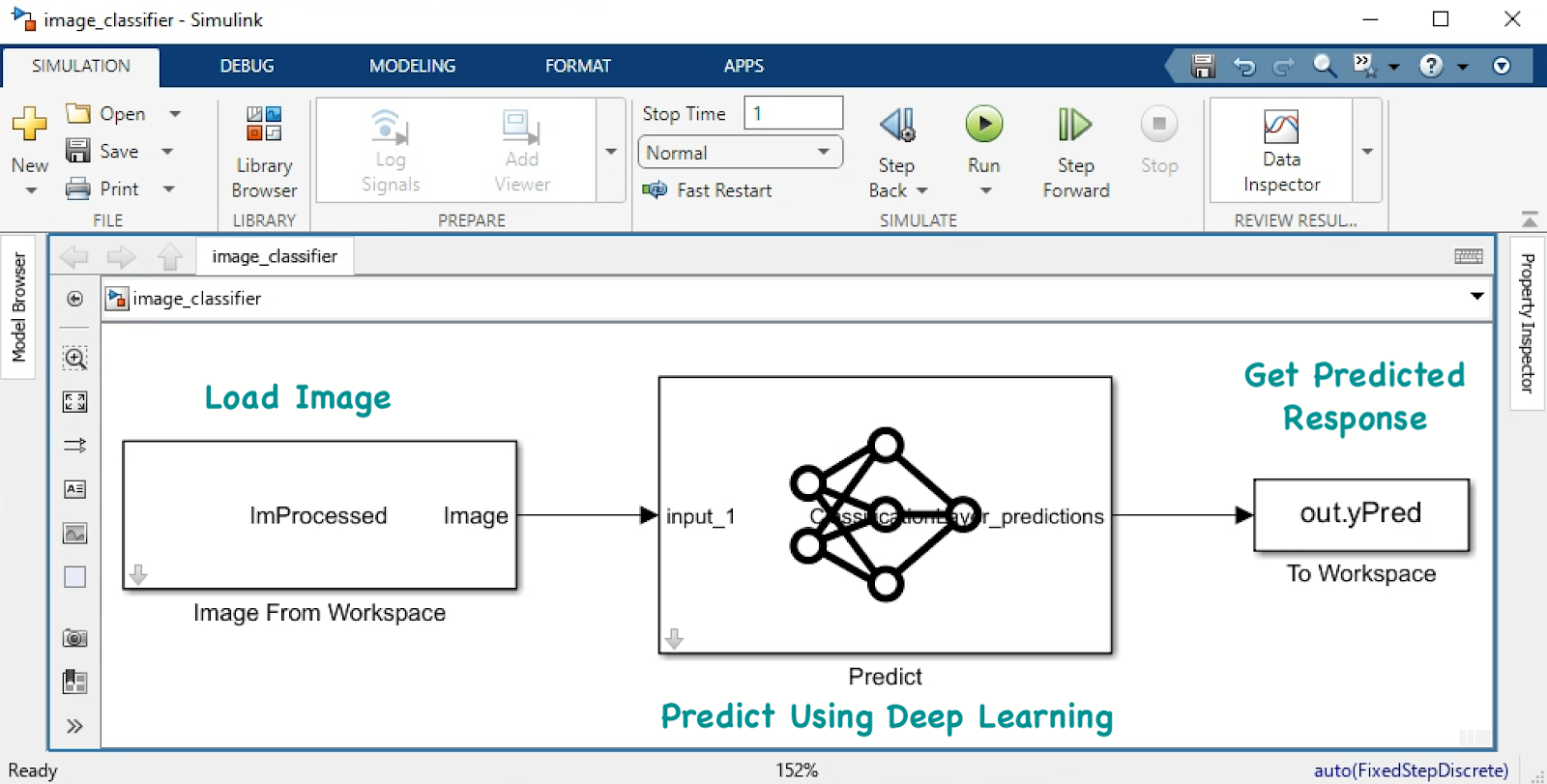Figure:Simple Simulink system for predicting image label

Of course, Simulink capabilities extend far beyond classifying an image of my dog after I gave him a bad haircut and trying to predict his breed. For example, you can use deep neural networks inside a Simulink model to perform lane and vehicle detection. To learn more, see Machine Learning with Simulink and NVIDIA Jetson.
Lane and vehicle detection in Simulink using deep learning

# Convert Model from MATLAB to TensorFlow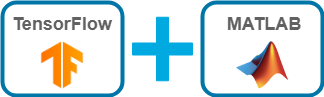You can convert a trained or untrained model from MATLAB to TensorFlow by using the MATLAB function exportNetworkToTensorFlow. In MATLAB, we refer to trained models as networks and to untrained models as layer graphs. The Pretrained Deep Neural Networks documentation page shows you all the options of how to get a pretrained network. You can alternatively create your own network.

#### Create Untrained Model

Create a bidirectional long short-term memory (BiLSTM) network to classify sequence data. An LSTM network takes sequence data as input and makes predictions based on the individual time steps of the sequence data.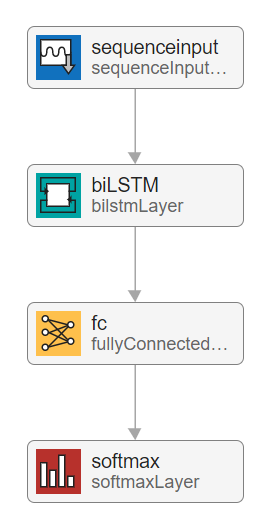Figure:Architecture of LSTM model
MATLAB code:

 ```inputSize = 12; numHiddenUnits = 100; numClasses = 9; layers = [ sequenceInputLayer(inputSize) bilstmLayer(numHiddenUnits,OutputMode="last") fullyConnectedLayer(numClasses) softmaxLayer]; lgraph = layerGraph(layers);```

To learn how to create the training data set for this model, see Export Untrained Layer Graph to TensorFlow. An important step is to permute the sequence data from the Deep Learning Toolbox ordering (CSN) to the TensorFlow ordering (NSC), where C is the number of features of the sequence, S is the sequence length, and N is the number of sequence observations. To learn more about the dimension ordering of the input data for different deep learning platforms, see Input Dimension Ordering.

#### Export Model to TensorFlow

Export the layer graph to TensorFlow. The exportNetworkToTensorFlow function saves the TensorFlow model in the Python package myModel.

MATLAB code:

 `exportNetworkToTensorFlow(lgraph,”myModel”)`

#### Train TensorFlow Model

Run the following code in Python to load the exported model from the Python package myModel. You can also compile and train the exported model in Python. To train the model, use the training data in training_data.mat that you previously created.

Python code:

 ```import myModel model = myModel.load_model()```

Python code:

 ```import scipy.io as sio data = sio.loadmat("training_data.mat") XTrain = data["XTrain"] YTrain = data["TTrain"]```

Compile and train model.

Python code:
 ```model.compile(optimizer = "adam", loss = "sparse_categorical_crossentropy", metrics=["accuracy"]) r = model.fit(XTrain, YTrain, epochs=100, batch_size=27)```

To learn more on how to export MATLAB models to TensorFlow, check out our blog post.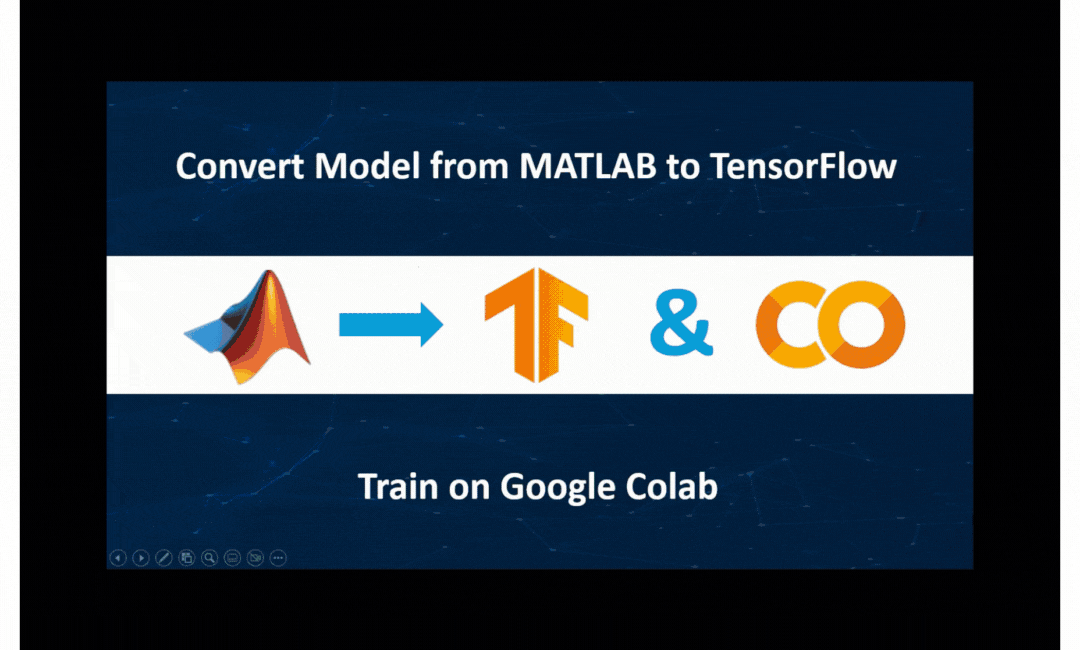Export untrained model from MATLAB to TensorFlow and train on Google Colab

# Run TensorFlow and MATLAB TogetherYou ‘ve seen so far how to convert models between TensorFlow and MATLAB. You also have the option to use TensorFlow and MATLAB together (run from the same environment) by either calling Python from MATLAB or calling MATLAB from Python. This way you can take advantage of the best capabilities from each environment by creating an integrated workflow.

For example, TensorFlow might offer newer models but you like MATLAB apps for labeling data, or you might want to train your TensorFlow model under multiple initial conditions using the Experiment Manager app (see example).

#### Call Python from MATLAB

Instead of importing a TensorFlow model into MATLAB you have the option to directly use the TensorFlow model in your MATLAB workflow by calling Python from MATLAB. You can access Python libraries by adding the py. prefix and execute any Python statement from MATLAB by using the pyrun function. For an example that shows how to call a TensorFlow model in MATLAB, see Image Classification in MATLAB Using TensorFlow.

A use case that this option might be useful is the following. You have created an object detection workflow in MATLAB. You want to quickly compare TensorFlow models to find the best suited model for your task before importing the best suited model into MATLAB. Call TensorFlow from MATLAB to run an inference test quickly.

#### Call MATLAB from Python

You can use MATLAB Engine API to call MATLAB from a Python environment and thus, integrate MATLAB tools and apps into your existing Python workflow. MATLAB is convenient for labeling and exploring data for domain-specific (e.g., radar, wireless, audio, and biomedical) signal processing using low-code apps. For an example, see our GitHub repo Co-Execution for Training a Speech Command Recognition System.

# Conclusion

The bottom line is that both TensorFlow and MATLAB offer excellent tools that enable applying deep learning to your application. MATLAB integrates with TensorFlow to take full advantage of these tools and enable access to hundreds of deep learning models. Choose between the interoperability features (convert models between TensorFlow and MATLAB, or use TensorFlow and MATLAB together) to create a deep learning workflow that bridges platforms and teams.

If you have questions about how, when, and why to use the described interoperability, email me at sparaske@mathworks.com. I would love to hear more about your workflow and discuss how working across deep learning platforms accelerates the application of deep learning to your domain.

Next postPosted by Sivylla Paraskevopoulou, Product Marketing Manager at MathWorksIn this blog post I will show you how to use TensorFlow™ with MATLAB® for deep learning applications. More specifically, I will show you how to convert pretrained TensorFlow models to MATLAB models, convert models from MATLAB to TensorFlow, and use MATLAB and TensorFlow together.These interoperability features, offered by MA…